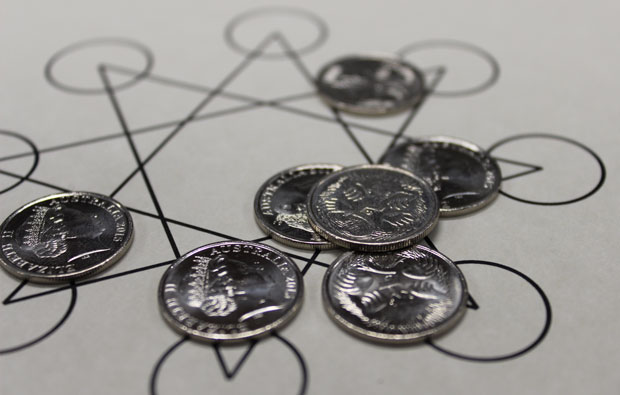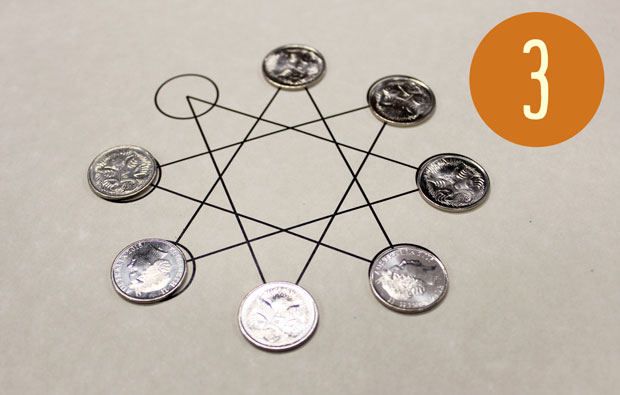What's newFive-cent star

By David, 1 August 2017 ActivityHere’s a tricky puzzle for you! An eight pointed star, and seven coins. Can you put all the coins onto the star?

The puzzle

1. The aim of the puzzle is to get all seven coins onto the points of the star. The circles are there to show you the positions in which you can put coins.
2.Putting a coin on the board is a two-step process:
• First, place the coin on an empty point.
• Then, slide the coin along one straight line to a different empty point.
3.That’s it! See if you can get all seven coins on the star. It’s harder than it looks!

What’s happening?

This puzzle can be tricky to get started. So here’s a mathematical way of breaking it down.

Start out by drawing a square. See if you can put three coins on the square, following the same rules. This puzzle is still hard, but it’s a bit easier to see what’s going on.

Next, scale the puzzle up. Try an octagon with eight points, and get seven coins on it.

Finally, go back to the star. The lines of the star go all over the place, but if you trace along them with your finger, you’ll uncover a hidden loop! Now, see if you can solve the puzzle.

1. The first move can be anywhere you like. Just remember where you put the coin originally.
2. The second coin needs to end up where you put the first coin originally. Look at that point, find the empty point connected to it, and place your coin there. Then slide it into place.
3. The third coin needs to end up where you placed the second originally. So once again, look at the lines and make the correct move.
4. Repeat this pattern and you’ll get all seven coins on the star!

If you’re after more hands-on activities for kids, subscribe to Double Helix magazine!Categories: